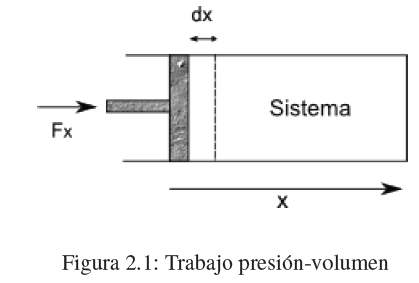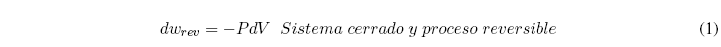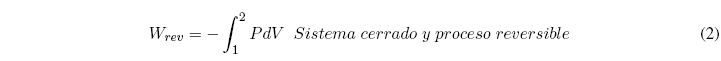As in classical mechanics, work is defined by the equation dw = F x dx,  where F x is the component of force F in the direction of motion and dx is the displacement. When F x and dx have the same sense, the work is positive, if they have  opposite directions the work is negative.Consider a cylinder with a piston acted on by a force Fx producing a piston displacement dx.  The work done on the system is given by the equation, dw =F x dx.  Let A be the area of the piston, the pressure exerted by the piston on the system will be, P=F x /A, substituting in the work expression, dw = PAdx.

The product Adx represents the volume variation produced by the movement of the piston dV=-Adx.  Substituting in dw we get:If we do work on the system (compression) dV<0 and the work is positive.  When the system does work on the surroundings (expansion) dV>0  work is negative.

So far we have only considered infinitesimal changes in volume dV.  The work done when going from a volume V 1 to V 2 is given by the sum of the infinite infinitesimal changes that allow the system to go from the first volume to the second.  In each of these steps the system changes by a volume dV and work is done –PdV.  The work on this volume change is given by the integral:What is a reversible process?  A reversible process is one in which every change with respect to an equilibrium state of the system is infinitesimal, for example, a finite volume change must be given by an infinite number of volume changes and the system remains close to equilibrium throughout. throughout the entire process.  This type of process can be reversed and the system will return to the same starting conditions.

In a PV diagram, the previous integral represents the area under the curve, and the work done will depend on the path followed to go from point 1 to point 2.  This means that work is not a state function. As can be seen in the graphs, the area under the curve is different depending on the trajectory followed to go from point 1 to 2.In short, work is the form of energy transfer between the system and the environment caused by a force that carries out a displacement.